Saturday, December 4, 2021
Home > CBSE Class 10 > Extra MCQ Questions for Class 10 Maths Chapter 12 Area Related to Circles

# Extra MCQ Questions for Class 10 Maths Chapter 12 Area Related to Circles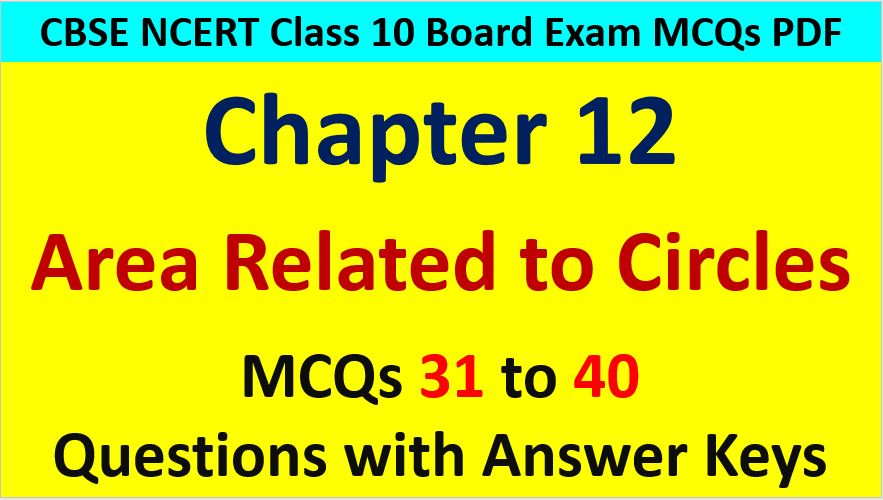Hi students, Welcome to AMBiPi (Amans Maths Blogs). In this article, you will get Extra MCQ Questions for Class 10 Maths Chapter 12 Area Related to Circles. You can download this PDF and save it in your mobile device or laptop etc.

Extra MCQ Questions for Class 10 Maths Area of Circles Question No 31:

In the figure below, DACB is a quadrant of a circle with centre O and radius 3.5 cm. If OD = 2, the area of the shaded region will beOption A : 12.125 cm

Option B : 6.125 cm

Option C : 8.5 cm

Option D : 10.25 cm

Option B : 6.125 cm

Extra MCQ Questions for Class 10 Maths Area of Circles Question No 32:

In the figure below, if AB = BC and BD = BF. If AC = 6, then find the area of the shaded region.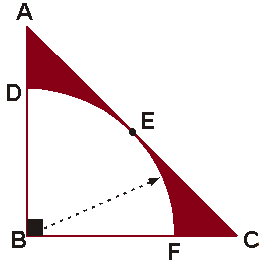Option A : 9(2 – π) / 4

Option B : 9(4 – π) / 4

Option C : 3(2 – π) / 4

Option D : 3(4 – π) / 4

Option A : 9(2 – π) / 4

Extra MCQ Questions for Class 10 Maths Area of Circles Question No 33:

In the figure below, if the side of the square is 2, then find the area of the shaded region.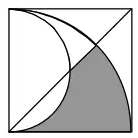Option A : (π – 2) / 4

Option B : (π – 2) / 2

Option C : (π + 2) / 4

Option D : (π + 2) / 2

Option C : (π + 2) / 4

Extra MCQ Questions for Class 10 Maths Area of Circles Question No 34:

In the figure below, ABCD is a square of side 14 cm. Semi-circle are drawn with each side of square as diameter. What is the area of the shaded region?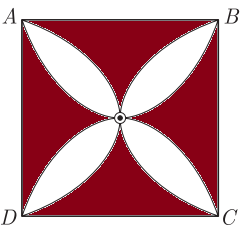Option A : 42 cm2

Option B : 84 cm2

Option C : 48 cm2

Option D : 96 cm2

Option B : 84 cm2

Extra MCQ Questions for Class 10 Maths Area of Circles Question No 35:

In the figure below, ABCD is a square of side 2, then find the area of the shaded region.Option A : (π – 2)

Option B : 2(π – 2)

Option C : (π – 1)

Option D : 2(π – 1)

Option B : 2(π – 2)

Extra MCQ Questions for Class 10 Maths Area of Circles Question No 36:

In the figure below, if the area of the shaded circle is 4π cm2, then find the area of circle.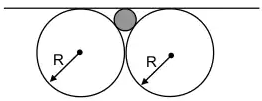Option A : 64π cm2

Option B : 32π cm2

Option C : 24π cm2

Option D : 16π cm2

Option A : 64π cm2

Extra MCQ Questions for Class 10 Maths Area of Circles Question No 37:

In the figure below, two arcs PAQ and PQB . Arc PAQ is a part of circle with centre O and radius OP while arc PBQ is a semi-circle drawn on PQ as diameter with centre M . If OP = PQ = 10 cm the area of shaded region will beOption A : 25(√3 – π/6) cm2

Option B : 50(√3 – π/6) cm2

Option C : 50(√3 + π/6) cm2

Option D : 25(√3 + π/6) cm2

Option A : 25(√3 – π/6) cm2

Extra MCQ Questions for Class 10 Maths Area of Circles Question No 38:

In the figure below, ABCD is a square of side 4 units. Find the area of the shaded region.Option A : 4 – π

Option B : 4

Option C : 8 – π

Option D : 8

Option B : 8

Extra MCQ Questions for Class 10 Maths Area of Circles Question No 39:

In the figure below, find the area of the shaded circle.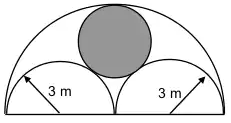Option A :

Option B :

Option C : 10π

Option D :

Option D : 4π

Extra MCQ Questions for Class 10 Maths Area of Circles Question No 40:

In the figure below, find the area of the shaded region.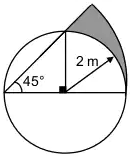Option A : (π – 1)

Option B : (π – 2)

Option C : (π – 3)

Option D : (π – 4)

Option B : (π – 2)

CBSE Class 10 Maths Chapterwise MCQs
1 : Real Numbers MCQ
| 1 to 10 | 11 to 20 | 21 to 30 | 31 to 40 | 41 to 50
2 : Polynomials MCQ
| 1 to 10 | 11 to 20 | 21 to 30 | 31 to 40 | 41 to 50
3 : Linear Equations MCQ
| 1 to 10 | 11 to 20 | 21 to 30 | 31 to 40 | 41 to 50
| 1 to 10 | 11 to 20 | 21 to 30 | 31 to 40 | 41 to 50
5 : Arithmetic Progression MCQ
| 1 to 10 | 11 to 20 | 21 to 30 | 31 to 40 | 41 to 50
6 : Triangles MCQ
| 1 to 10 | 11 to 20 | 21 to 30 | 31 to 40 | 41 to 50
7 : Coordinate Geometry MCQ
| 1 to 10 | 11 to 20 | 21 to 30 | 31 to 40 | 41 to 50
8 : Introduction to Trigonometry MCQ
| 1 to 10 | 11 to 20 | 21 to 30 | 31 to 40 | 41 to 50
9 : Application of Trigonometry MCQ
| 1 to 10 | 11 to 20 | 21 to 30 | 31 to 40 | 41 to 50
10 : Circles MCQ
| 1 to 10 | 11 to 20 | 21 to 30 | 31 to 40 | 41 to 50
11 : Constructions MCQ
| 1 to 10 | 11 to 20
12 : Area Related to Circles MCQ
| 1 to 10 | 11 to 20 | 21 to 30 | 31 to 40 | 41 to 50
13 : Surface Area & Volume MCQ
| 1 to 10 | 11 to 20 | 21 to 30 | 31 to 40 | 41 to 50
14 : Statistics MCQ
| 1 to 10 | 11 to 20 | 21 to 30 | 31 to 40 | 41 to 50
15 : Probability MCQ
| 1 to 10 | 11 to 20 | 21 to 30 | 31 to 40 | 41 to 50
16 : Previous Year Maths MCQs from CBSE Board Class 10
| 1 to 100
AMBiPi
error: Content is protected !!